# Heat and Temperature Explained | Examples

Want create site? Find Free WordPress Themes and plugins.

Heat and temperature are different parameters. Heat is energy stored in a body, whereas temperature is the potential of that heat, just as voltage is the potential in an electric circuit. A candle flame has a temperature which is high enough to burn fingers, but a person can quickly snuff out a candle flame between their fingertips without burning themselves because the energy in the candle flame is small.

Heat energy flows from bodies with high temperatures to bodies with low temperatures, due to the potential difference in temperature.

Temperature

When heat is stored in a body, the temperature rises according to the ‘specific heat capacity’ of that body to absorb heat. When a material reaches a specific temperature, such as the temperature at which it melts or turns into a gas, more energy is required to convert the material from one state to another, and so the temperature remains the same until all the material has been converted. These two temperatures are known as the ‘specific heat of fusion’ (melting) and the ‘specific heat of vaporization’ (evaporating or becoming a gas).

These two temperatures form two defining points for most temperature scales.

The Celsius or Centigrade scale has a lower point of 0ºC, taken as the point when pure water freezes, and a higher point of 100ºC, when pure water boils into a gas (vapor or steam).

The SI unit of temperature is the Kelvin. (Note that the word degree is not used for the Kelvin scale.) One Kelvin is equal to 1ºC but, instead of the lower temperature point being 0ºC, Kelvin is an absolute scale which means that 0 K is the temperature at which a body has no energy at all.

Lord Kelvin (William Thomson) discovered that all materials share the same zero energy or absolute zero temperature of –273.16ºC, as predicted in graphs that he produced. That temperature is known as absolute zero or zero Kelvin (0 K).

Heat

Heat energy is the same as energy in other systems, and the unit for heat energy is the same SI unit as used for mechanical and electrical energy units (the joule).

Heat can be stored in a body of material or transferred from one body to another when a suitable temperature difference exists and a path is available for the heat to flow across.

Specific Heat Capacity

When equal masses of different materials receive equal quantities of heat energy, different temperature increases may result. This is a result of the property of a material known as its specific heat capacity, some typical values of which are given in Table 1. Note that the specific heat capacity of water (4180 J/kgK) is high compared to that of many other materials.

Table 1 Specific Heat Capacities of Common Materials

 Material Specific heat capacity*(in joules/ kgK) Water 4187 Aluminum 900 Copper 390 Gold 130 Silver 230 Lead 130 Zinc 390

The specific heat capacity of a material is defined as the quantity of heat energy required to raise the temperature of a mass of one kilogram by one Kelvin (joules/kgK).

The amount of energy that is contained in a body of material has been found to depend on three parameters: the mass of material in the body (M), the type of material (the specific heat capacity of the material — c) and the temperature of the material (T).To calculate the energy required to change the temperature from one value to another, the same formula can be used but with t to represent the change in temperature measured in ºC instead of absolute temperature (K).

Remember that a change in temperature measured in ºC is the same as a change in temperature measured in Kelvin as one ºC equals one K.

The usual type of problem that an electrician might need to solve involves calculating the energy and/or power required to change the temperature of a body from one value to another. One example is in raising a quantity of water to boiling point.

Many processes, industrial and domestic, are based on boiling water. Example 2 is representative of such a calculation. Note that the value of c is given as 4180 but at other times is as high as 4187. The value changes with barometric pressure and therefore with height above sea level.

Example1

An urn containing 18 liters of water is heated from room temperature (20ºC) to boiling point (100ºC).

 (a) How much heat energy must be delivered to the urn? (b) How much power would be required to boil the water in one hour?

(C for water = 4180 J/kgK)

Step 1. Change in temperature: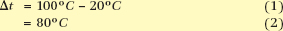Step 2. Heat energy:Step 3. Power required:Another calculation, less common but nevertheless valuable, is to calculate the temperature of a mass of metal in some heat transfer process e.g. an electronic heat sink. Example 3 is typical of such a calculation. The specific heat values can be taken from Table 1.

Example 2

A copper and an aluminum block (both of mass 1.5 kg) have 2 kJ of heat energy added to each. If the initial temperature was 25ºC, calculate the final temperature for each block.

Step 1. By transposition:Step 2. Change in temperature for the copper: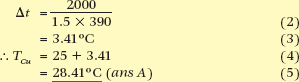Step 3. Change in temperature for the aluminum: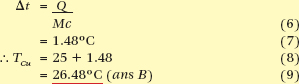Heat Transfer

Heat energy moves from high to low temperature levels, and the rate of energy transfer depends on the temperature difference between the two levels. That is, a higher temperature difference gives a faster heat transfer rate than a lower temperature difference.

There are three recognized processes of heat transfer.

 1 Conduction. As a material rises in temperature, the molecules and their associated electrons gain energy. This extra energy is transferred through the material by means of collisions between electrons and atoms. 2 Convection is the transfer of heat energy from one place to another by the actual movement of the material. For example, as a bowl of water is heated, the molecules near the heat source are energized and rise through the water, while colder molecules move into their place. The currents generated in the water are called convection currents. 3 Radiation. Heat energy is transformed into radiant energy, which is electromagnetic radiation, just like light or radio waves. In this form, the energy can travel considerable distances and can also travel freely in a vacuum. When the radiation reaches another body of material, the radiant energy adds to the heat energy of that material.

Thermal Conductivity

The rate of heat conduction through a material is joules per second, and therefore is measured in watts. The rate of heat flow depends on:

 1 temperature difference (gradient) across the material 2 cross-sectional area of transfer path 3 length of transfer path 4 Type of material.

The best conductors of heat are metals such as aluminum and copper, which are also good electrical conductors. Poor thermal conductors such as wood, glass and ceramics offer higher opposition to heat energy flow and are therefore considered thermal insulators.

Thermal conductivity is the ability of a material to transmit heat by conduction. For any one material this is a constant value and is often called the ‘k-factor’.

When heat is being generated within a material, such as a conductor with an electric current flowing through it, the temperature of the conductor begins to rise. Heat energy passes through the insulation at a rate determined by the insulation’s thermal conductivity. The heat energy is transferred either to the air, where it is convected away, or to a surface, where it conducts away. Some heat is also given off by radiation.

The temperature of the environment surrounding the conductor is known as the ‘ambient temperature’. When the heat energy being dissipated is equal to that being generated, the temperature of the conductor stabilizes at some temperature above ambient.

Heat energy losses can be an advantage, for example, when there is a need to dissipate heat to avoid melting the insulation or even the conductors themselves.

In the case of a hot-water storage heater, heat loss leads to lower efficiency and higher operating costs, so in this case heat loss is a disadvantage which is therefore to be avoided.

To reduce losses and retain heat energy, manufacturers wrap the hot-water container in a material which has poor thermal conductivity, that is, a good thermal insulating property. Plastic foams, fiberglass and insulating wool are examples of thermal insulators.

Electrical Heating

Most electrical appliances rely on the principle that current flowing through a resistor consumes electrical energy and transforms it into heat energy. The heat energy that results from electric current flow is determined by the power used by the appliance multiplied by the length of time it is used for.

Power is calculated from voltage multiplied by current, but when the voltage is constant, for example 230 volts, and the resistance is known, such as in a fixed heating element or the resistance of the wiring itself, power is calculated from I2R.

The energy generated, therefore, is I2R multiplied by time or I2RT. In fact, the heat losses in an electrical machine or wiring system are often described as ‘I2RT’ losses.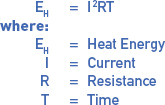Example 4 shows a calculation using this formula. Remember to convert minutes into seconds. This is a common mistake students make in exams. There are 60 seconds in a minute and 3600 seconds in an hour (60 × 60).

Example 3

An electric motor is stalled, i.e. is not running, but with 32 volts across the terminals and 2.5 Ω winding resistance takes 1.5 minutes for the protection to operate. How much heat energy is generated?

Step 1. Find Current flow:Step 2. Calculate energy: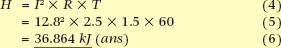Example 4

A farmer uses 1 mm2 wire to run a 10 amp pump motor 50 meters from his shed. If the total resistance of the wire is 1.7 ohms, how much energy is lost per hour?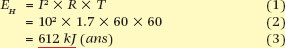Did you find apk for android? You can find new Free Android Games and apps.